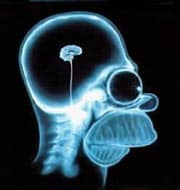# Science without sense…double nonsense

## Size and power

This post is also available in: Spanish

Two associated qualities. And very enviable too. Especially when it comes to scientific studies (what were you thinking about?). Although there’re more factors involved, as we’ll see in a moment.

Let’s suppose we are measuring the mean of a variable in two samples to find out if there’re differences between them. We know that, just by random sampling, the results of the two samples will be different but we’ll want to know if that difference is wide enough to allow us to suppose they are actually different.

To find it out we make a hypothesis testing using the appropriate statistical. In our case, let’s suppose we do a Student t test. We calculate the value of our t and estimate its probability. Most of statistical, included t, follow a specific frequency or probability distribution. These distributions are generally bell-shape, more or less symmetrical and centered on certain value. Thus, values near the center are more likely to occur, while those in the extremes edges are less likely. By convention, when this probability is less than 5% we consider the occurrence of that value of the parameter measured unlikely to happen.

But of course, unlikely is not synonymous with impossible. It may be that, by chance, we have choose a sample that is not centered on the same value as the reference population, so the value happens in spite of its low probability of happening in this population.

And this is important because it can lead to errors in our conclusions. Remember that when we have two values to compare we establish the null hypothesis (H0) that the two values are equivalent, and that any difference is due to a random sampling error. Then, if we know its frequency distribution, we can calculate the probability of that difference occurring by chance. Finally, if it is less than 5% we’ll consider unlikely for it to be fortuitous and we’ll reject H0: the difference is not the result of chance and there’s a real effect or a real difference.

But again, unlikely is not impossible. If we have the misfortune of having chosen a biased sample to the population, we could reject the null hypothesis without having a real effect and commit a type 1 error.

Conversely, if the probability is greater than 5% we will not be able to reject H0 and we will say that the difference is due to chance. But here’s a little concept hue that is important to consider. The null hypothesis is only falsifiable. This means that we can reject it, but not affirm it. When we cannot reject it, if we assume it’s true we’ll run the risk of not detecting a trend that really exist. This is the type 2 error.

Usually we are more interested in accepting theories as safely as possible, so we look for low type 1 error probabilities, usually 5%. This is called the alpha value. But the two types of errors are interlinked, so a very low alpha compels us to accept a higher type 2 error (or beta) probability, generally 20%.

The reciprocal value of beta is what is called the power of the study (1-beta). This power is the probability of detecting an effect, given that it really exists, or put it in other words, the probability of not committing a type 2 error.

To understand the factors involved with the study power, will you let me pester you with a little equation:

SE represents the standard error. Being it in the numerator implies that the lower SE (the more subtle the difference) the lower the power of the study to detect the effect. The same applies to the sample size (n) and alpha: the larger the sample and the higher the significance that we tolerate (with increased risk of type 1 error), the greater the power of the study. Finally, s is the standard deviation: the more variability is in the population, the lower the power of the study.

The utility of the above equation is that we can solve is to obtain the sample size in the following way:

n∝((1-β)×σ^2)/(SE×α)

With this formula we can calculate the sample size we need to get the desired power we choose. Beta is usually set at 0.8 (80%). SE and s are obtained from pilot studies or previous data or regulations and, if they don’t exist, they are set by the researcher. Finally, as we have already mentioned, alpha is usually set at 0.05 (5%), although if we are very afraid of committing a type 1 error we can set it at 0.01.

Closing this post, I would like to draw your attention to the relationship between n and alpha in the first equation. Notice that the power doesn’t change if we increase sample size and concomitantly diminish the significance level. This leads to the situation that, sometimes, to obtain statistical significance is only a matter of increasing enough the sample size. It is therefore essential to assess the clinical relevance of the results and not just its p-values. But that’s another story…

This site uses Akismet to reduce spam. Learn how your comment data is processed.